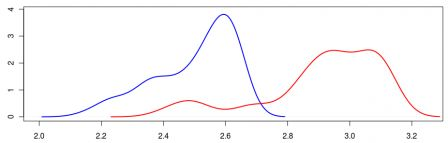Want to share your content on R-bloggers? click here if you have a blog, or here if you don't.It is common to look at best time at the Marathon. Or perhaps the distribution of the top100, as done by John Myles White on his blog here (data can be found there), as the graph below, with the density of the time for the first 100 men (in blue) and the first 100 women (in red).Hence, men and women are different and men run faster than women. So what…?
A lot of people run those marathon. Almost 40,000 ran (and finished) the one in New York City. Instead of 100, shouldn’t we keep more runners, e.g the top 5% ?
The other point is that it might be interesting to correct (or control) with the age. If men and women running the marathon do not have the same age, it might be an explanation for that difference.
Unfortunately, to run a quantile regression properly I need the entire dataset, with 40,000 runners. And on the website of the NY Marathon (here) it is possible to extract some information, but not the entire dataset (and my standard R robots did not work here). So I managed to extract the “worst” 10% runners in 2008, and run a quantile regression to get the 95% quantile regression curve, using the idea mentioned here.I got the 95% quantile curve for men (in blue) and women (in red), and similarly for top runner, namely the 5% quantile curve.On those graphs, if we exclude runners under 22, quantile curves for men are usually below the ones for women. Which confirms the first order dominance mentioned by John,
For those willing to play, here is the code (it is dirty since my dataset was dirty)
```dataend=read.table("/home/arthur/NY2008-top.csv",header=FALSE,sep="t")
nrow(dataend)
I=dataend[,5]
I[is.na(dataend[,5])]=dataend[is.na(dataend[,5]),6]
q=which(substr(as.character(I),1,1)%in%c("M","F"))
I=I[q]
age=as.numeric(substr(as.character(I),2,3))
sex=substr(as.character(I),1,1)=="M"
tp=as.character(dataend[,45])
i=which(tp=="");tp[i]=as.character(dataend[i,44])
i=which(tp=="");tp[i]=as.character(dataend[i,43])
i=which(tp=="");tp[i]=as.character(dataend[i,42])
tp=tp[q]
temps=((as.numeric(substr(tp,1,1))+as.numeric(substr(tp,3,4))/60)*26.21875)/60
propend=length(tp)/n2008Men
base=data.frame(age,temps)
base=base[sex==TRUE,]
reg=rq(temps~bs(age,7),data=base,tau=.05/propend)
u=seq(20,80)
bu=data.frame(age=u)
y2008m=predict(reg,newdata=bu)
propend=length(tp)/n2008Women
base=data.frame(age,temps)
base=base[sex==FALSE,]
reg=rq(temps~bs(age,5),data=base,tau=.05/propend)
u=seq(20,80)
bu=data.frame(age=u)
y2008w=predict(reg,newdata=bu)```
But looking at archives (here 1983 compared with 2008) I noticed something strange. First, if we compare number of runners (here starters),
 Men Women 1983 12,838 2,553 2008 25,669 13,163
it looks like more and more women run the marathon nowadays. So this might have an impact on the results,On the graph above, the have a strong line for 2008 and a thin one for 1983, and again men in blue and women in red. It looks like fast runners run as fast as before, but since there are more and more runners, the quantiles are going up, And by that time, women who ran the marathon were performing extremely well compared with men.
Anyway, this is now really a statistical analysis, but more a “how to say something when you cannot access a database entirely“. But I still hope someday I will…